Anúncio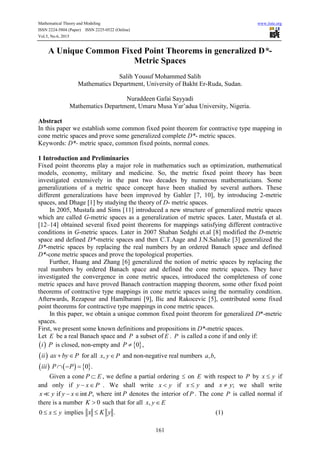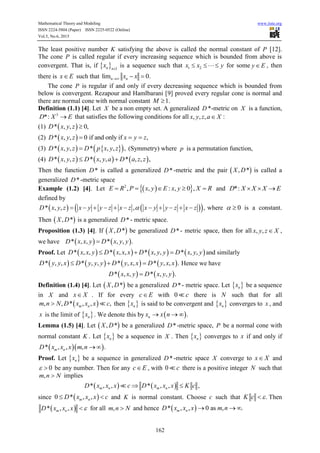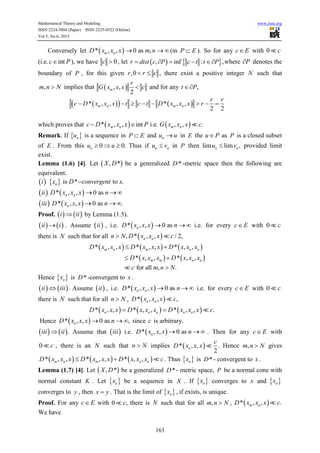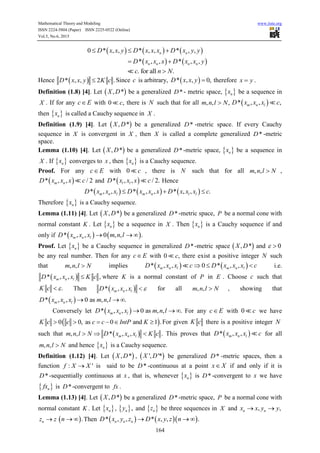Anúncio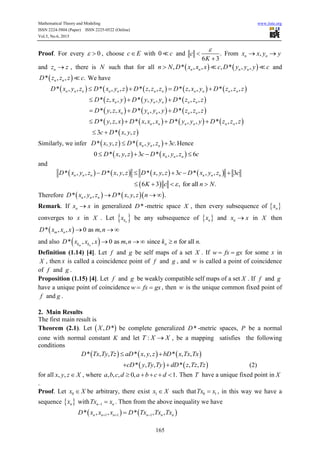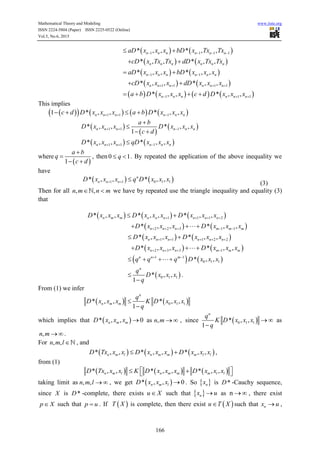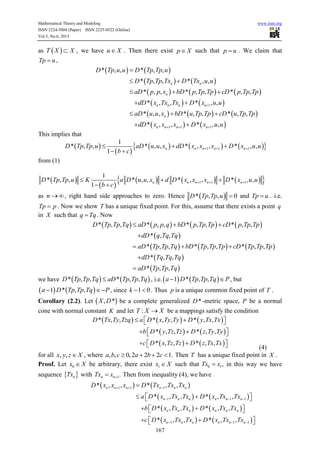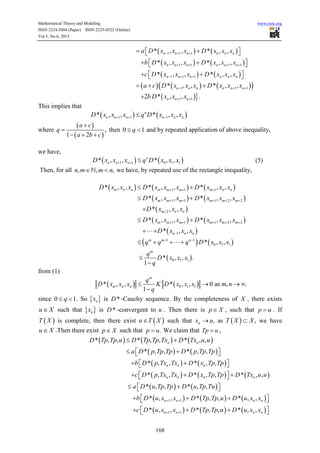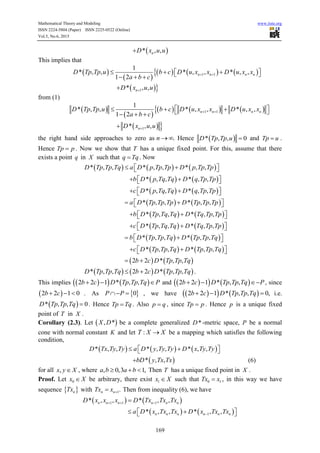Anúncio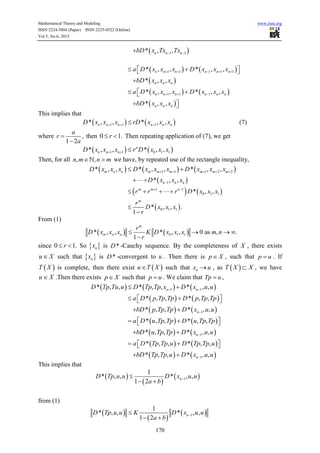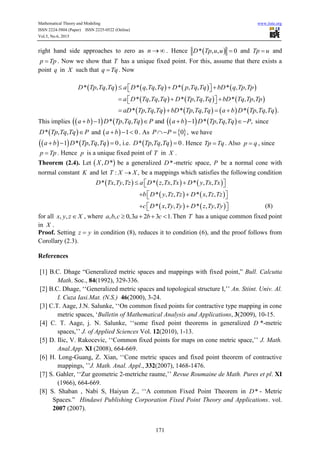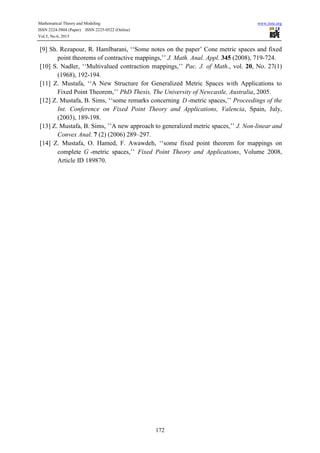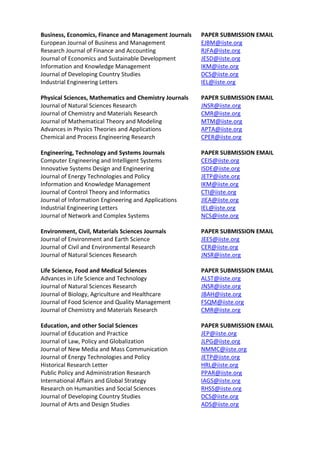Próximos SlideShares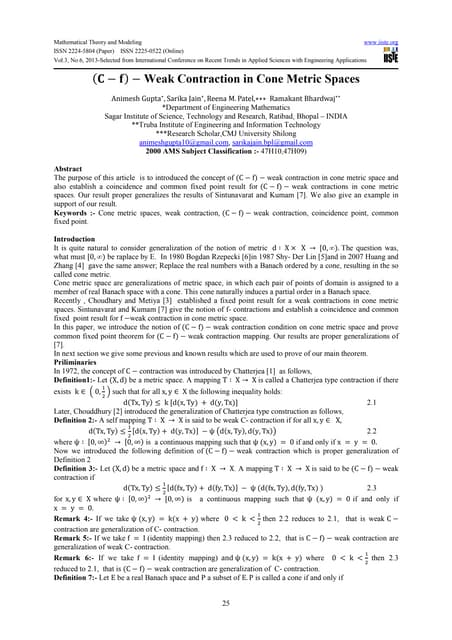(C f)- weak contraction in cone metric spaces
Carregando em ... 3
1 de 14
Anúncio

### A unique common fixed point theorems in generalized d

1. Mathematical Theory and Modeling www.iiste.org ISSN 2224-5804 (Paper) ISSN 2225-0522 (Online) Vol.5, No.6, 2015 161 A Unique Common Fixed Point Theorems in generalized D*- Metric Spaces Salih Yousuf Mohammed Salih Mathematics Department, University of Bakht Er-Ruda, Sudan. Nuraddeen Gafai Sayyadi Mathematics Department, Umaru Musa Yar’adua University, Nigeria. Abstract In this paper we establish some common fixed point theorem for contractive type mapping in cone metric spaces and prove some generalized complete D*- metric spaces. Keywords: D*- metric space, common fixed points, normal cones. 1 Introduction and Preliminaries Fixed point theorems play a major role in mathematics such as optimization, mathematical models, economy, military and medicine. So, the metric fixed point theory has been investigated extensively in the past two decades by numerous mathematicians. Some generalizations of a metric space concept have been studied by several authors. These different generalizations have been improved by Gahler [7, 10], by introducing 2-metric spaces, and Dhage  by studying the theory of D- metric spaces. In 2005, Mustafa and Sims  introduced a new structure of generalized metric spaces which are called G-metric spaces as a generalization of metric spaces. Later, Mustafa et al. [12–14] obtained several fixed point theorems for mappings satisfying different contractive conditions in G-metric spaces. Later in 2007 Shaban Sedghi et.al  modified the D-metric space and defined D*-metric spaces and then C.T.Aage and J.N.Salunke  generalized the D*-metric spaces by replacing the real numbers by an ordered Banach space and defined D*-cone metric spaces and prove the topological properties. Further, Huang and Zhang  generalized the notion of metric spaces by replacing the real numbers by ordered Banach space and defined the cone metric spaces. They have investigated the convergence in cone metric spaces, introduced the completeness of cone metric spaces and have proved Banach contraction mapping theorem, some other fixed point theorems of contractive type mappings in cone metric spaces using the normality condition. Afterwards, Rezapour and Hamlbarani , Ilic and Rakocevic , contributed some fixed point theorems for contractive type mappings in cone metric spaces. In this paper, we obtain a unique common fixed point theorem for generalized D*-metric spaces. First, we present some known definitions and propositions in D*-metric spaces. Let E be a real Banach space and P a subset of E . P is called a cone if and only if:  i P is closed, non-empty and  0 ,P   ii ax by P  for all ,x y P and non-negative real numbers , ,a b      0 .iii P P   Given a cone P E , we define a partial ordering  on E with respect to P by x y if and only if y x P  . We shall write x y if x y and ;x y we shall write if int ,x y y x P  where int P denotes the interior of P . The cone P is called normal if there is a number 0K  such that for all ,x y E 0 x y  implies .x K y (1)
2. Mathematical Theory and Modeling www.iiste.org ISSN 2224-5804 (Paper) ISSN 2225-0522 (Online) Vol.5, No.6, 2015 162 The least positive number K satisfying the above is called the normal constant of P . The cone P is called regular if every increasing sequence which is bounded from above is convergent. That is, if   1n n x  is a sequence such that 1 2x x y    for some y E , then there is x E such that lim 0.n nx x   The cone P is regular if and only if every decreasing sequence which is bounded from below is convergent. Rezapour and Hamlbarani  proved every regular cone is normal and there are normal cone with normal constant 1.M  Definition (1.1) . Let X be a non empty set. A generalized D *-metric on X is a function, 3 *:D X E that satisfies the following conditions for all , , ,x y z a X : (1)  * , , 0,D x y z  (2)  * , , 0 if and only if ,D x y z x y z   (3)     * , , * , , ,D x y z D p x y z (Symmetry) where p is a permutation function, (4)      * , , * , , * , , ,D x y z D x y a D a z z  Then the function *D is called a generalized *D -metric and the pair  , *X D is called a generalized *D -metric space Example (1.2) . Let   2 , , : , 0 ,E R P x y E x y X R     and *:D X X X E   defined by     * , , , ,D x y z x y y z x z x y y z x z           where 0  is a constant. Then  , *X D is a generalized *D - metric space. Proposition (1.3) . If  , *X D be generalized *D - metric space, then for all , ,x y z X , we have    * , , * , , .D x x y D x y y Proof. Let        * , , * , , * , , * , ,D x x y D x x x D x y y D x y y   and similarly        * , , * , , * , , * , , .D y y x D y y y D y x x D y x x   Hence we have    * , , * , , .D x x y D x y y Definition (1.4) . Let  , *X D be a generalized *D - metric space. Let  nx be a sequence in X and x X . If for every c E with 0 c there is N such that for all  , , * , , ,m nm n N D x x x c then  nx is said to be convergent and  nx converges to x , and x is the limit of  nx . We denote this by  .nx x n   Lemma (1.5) . Let  , *X D be a generalized *D -metric space, P be a normal cone with normal constant K . Let  nx be a sequence in X . Then  nx converges to x if and only if   * , , , .m nD x x x m n   Proof. Let  nx be a sequence in generalized *D -metric space X converge to x X and 0  be any number. Then for any c E , with 0 c there is a positive integer N such that ,m n N implies    * , , * , , ,m n m nD x x x c D x x x K c  since  0 * , ,m nD x x x c  and K is normal constant. Choose c such that .K c  Then  * , ,m nD x x x  for all ,m n N and hence  * , , 0 as , .m nD x x x m n  
3. Mathematical Theory and Modeling www.iiste.org ISSN 2224-5804 (Paper) ISSN 2225-0522 (Online) Vol.5, No.6, 2015 163 Conversely let  * , , 0 as ,m nD x x x m n  (in P E ). So for any c E with 0 c (i.e. intc P ), we have 0c  , let    , inf : ,r dist c P c t t P     where P denotes the boundary of P , for this given ,0 ,r r c  there exist a positive integer N such that ,m n N implies that  , , 2 m r G x x x c and for any ,t P     * , , * , , 2 2 m n m n r r c D x x x t c t D x x x r        which proves that    * , , int i.e. , , .m n m nc D x x x P G x x x c  Remark. If  nu is a sequence in P E and nu u in E the u P as P is a closed subset of E . From this 0 0.nu u   Thus if n nu v in P then lim lim ,n nu v provided limit exist. Lemma (1.6) . Let  , *X D be a generalized *D -metric space then the following are equivalent.     is * to .ni x D convergent x    * , , 0 asn nii D x x x n      * , , 0 as .niii D x x x n   Proof.    i ii by Lemma (1.5).    ii i . Assume  ii , i.e.  * , , 0 asnD x x x n   i.e. for every c E with 0 c there is N such that for all  , * , , / 2,n nn N D x x x c      * , , * , , * , ,m n m n nD x x x D x x x D x x x     * , , * , ,m m n nD x x x D x x x  for all , .c m n N Hence  nx is *D -convergent to x .    .ii iii Assume  ii , i.e.  * , , 0 asn nD x x x n   i.e. for every c E with 0 c there is N such that for all n N ,  * , , ,n nD x x x c      * , , * , , * , , .n n n n nD x x x D x x x D x x x c  Hence  * , , 0 as ,nD x x x n   since c is arbitrary.    .iii ii Assume that  iii i.e.  * , , 0 asnD x x x n   . Then for any c E with 0 c , there is an N such that n N implies  * , , . 2 n c D x x x Hence ,m n N gives      * , , * , , * , ,m n m n nD x x x D x x x D x x x c  . Thus  nx is *D - convergent to x . Lemma (1.7) . Let  , *X D be a generalized *D - metric space, P be a normal cone with normal constant K . Let  nx be a sequence in X . If  nx converges to x and  nx converges to y , then x y . That is the limit of  nx , if exists, is unique. Proof. For any c E with 0 ,c there is N such that for all ,m n N ,  * , , .m nD x x x c We have
4. Mathematical Theory and Modeling www.iiste.org ISSN 2224-5804 (Paper) ISSN 2225-0522 (Online) Vol.5, No.6, 2015 164      0 * , , * , , * , ,n nD x x y D x x x D x y y      * , , * , ,n n n nD x x x D x x y  . for all .c n N Hence  * , , 2 .D x x y K c Since c is arbitrary,  * , , 0,D x x y  therefore x y . Definition (1.8) . Let  , *X D be a generalized *D - metric space,  nx be a sequence in X . If for any c E with 0 ,c there is N such that for all , , ,m n l N  * , , ,m n lD x x x c then  nx is called a Cauchy sequence in X . Definition (1.9) . Let  , *X D be a generalized *D -metric space. If every Cauchy sequence in X is convergent in X , then X is called a complete generalized *D -metric space. Lemma (1.10) . Let  , *X D be a generalized *D -metric space,  nx be a sequence in X . If  nx converges to x , then  nx is a Cauchy sequence. Proof. For any c E with 0 c , there is N such that for all , ,m n l N ,  * , , / 2m nD x x x c and  * , , / 2.l lD x x x c Hence      * , , * , , * , , .m n l m n l lD x x x D x x x D x x x c   Therefore  nx is a Cauchy sequence. Lemma (1.11) . Let  , *X D be a generalized *D -metric space, P be a normal cone with normal constant K . Let  nx be a sequence in X . Then  nx is a Cauchy sequence if and only if    * , , 0 , , .m n lD x x x m n l   Proof. Let  nx be a Cauchy sequence in generalized *D -metric space  , *X D and 0  be any real number. Then for any c E with 0 ,c there exist a positive integer N such that , ,m n l N implies    * , , 0 * , ,m n l m n lD x x x c D x x x c   i.e.  * , , ,m n lD x x x K c where K is a normal constant of P in E . Choose c such that .K c  Then  * , ,m n lD x x x  for all , ,m n l N , showing that  * , , 0 as , , .m n lD x x x m n l   Conversely let  * , , 0 as , , .m n lD x x x m n l   For any c E with 0 c we have  0 0, as 0 and 1 .K c c c c IntP K      For given K c there is a positive integer N such that  , , * , , .m n lm n l N D x x x K c   This proves that  * , ,m n lD x x x c for all , ,m n l N and hence  nx is a Cauchy sequence. Definition (1.12) . Let  , *X D ,  ', '*X D be generalized *D -metric spaces, then a function : 'f X X is said to be *D -continuous at a point x X if and only if it is *D -sequentially continuous at x , that is, whenever  nx is *D -convergent to x we have  nfx is *D -convergent to fx . Lemma (1.13) . Let  , *X D be a generalized *D -metric space, P be a normal cone with normal constant K . Let  nx ,  ,ny and  nz be three sequences in X and , ,n nx x y y  nz z  .n   Then     * , , * , , .n n nD x y z D x y z n  
5. Mathematical Theory and Modeling www.iiste.org ISSN 2224-5804 (Paper) ISSN 2225-0522 (Online) Vol.5, No.6, 2015 165 Proof. For every 0  , choose c E with 0 c and . 6 3 c K    From ,n nx x y y  and nz z , there is N such that for all    , * , , , * , ,n n n nn N D x x x c D y y y c and  * , , .n nD z z z c We have          * , , * , , * , , * , , * , ,n n n n n n n n n n nD x y z D x y z D z z z D z x y D z z z         * , , * , , * , ,n n n n nD z x y D y y y D z z z        * , , * , , * , ,n n n n nD y z x D y y y D z z z          * , , * , , * , , * , ,n n n n n nD y z x D x x x D y y y D z z z     3 * , ,c D x y z  Similarly, we infer    * , , * , , 3 .n n nD x y z D x y z c  Hence    0 * , , 3 * , , 6n n nD x y z c D x y z c    and        * , , * , , * , , 3 * , , 3n n n n n nD x y z D x y z D x y z c D x y z c      6 3 , for all .K c n N    Therefore     * , , * , , .n n nD x y z D x y z n   Remark. If nx x in generalized *D -metric space X , then every subsequence of  nx converges to x in X . Let  nkx be any subsequence of  nx and nx x in X then  * , , 0 as ,m nD x x x m n   and also  * , , 0 as , since for all .m nk k nD x x x m n k n n    Definition (1.14) . Let f and g be self maps of a set X . If w fx gx  for some x in X , then x is called a coincidence point of f and g , and w is called a point of coincidence of f and g . Proposition (1.15) . Let f and g be weakly compatible self maps of a set X . If f and g have a unique point of coincidence w fx gx  , then w is the unique common fixed point of f and g . 2. Main Results The first main result is Theorem (2.1). Let  , *X D be complete generalized *D -metric spaces, P be a normal cone with normal constant K and let :T X X , be a mapping satisfies the following conditions      * , , * , , * , ,D Tx Ty Tz aD x y z bD x Tx Tx     * , , * , ,cD y Ty Ty dD z Tz Tz  (2) for all , ,x y z X , where , , , 0, 1.a b c d a b c d     Then T have a unique fixed point in X . Proof. Let 0x X be arbitrary, there exist 1x X such that 0 1Tx x , in this way we have a sequence  nx with 1n nTx x  . Then from the above inequality we have    1 1 1* , , * , ,n n n n n nD x x x D Tx Tx Tx  
6. Mathematical Theory and Modeling www.iiste.org ISSN 2224-5804 (Paper) ISSN 2225-0522 (Online) Vol.5, No.6, 2015 166    1 1 1 1* , , * , ,n n n n n naD x x x bD x Tx Tx        * , , * , ,n n n n n ncD x Tx Tx dD x Tx Tx     1 1* , , * , ,n n n n n naD x x x bD x x x      1 1 1 1* , , * , ,n n n n n ncD x x x dD x x x            1 1 1* , , * , ,n n n n n na b D x x x c d D x x x      This implies         1 1 11 * , , * , ,n n n n n nc d D x x x a b D x x x           1 1 1* , , * , , 1 n n n n n n a b D x x x D x x x c d           1 1 1* , , * , ,n n n n n nD x x x qD x x x   where  1 a b q c d     , then0 1q  . By repeated the application of the above inequality we have    1 1 0 1 1* , , * , ,n n n nD x x x q D x x x   (3) Then for all , ,n m n m  we have by repeated use the triangle inequality and equality (3) that      1 1 1 2* , , * , , * , ,n m m n n n n n nD x x x D x x x D x x x        2 2 3 1 1* , , * , ,n n n m m mD x x x D x x x         1 1 1 2 2* , , * , ,n n n n n nD x x x D x x x         2 3 3 1* , , * , ,n n n m m mD x x x D x x x        1 1 0 1 1* , ,n n m q q q D x x x      0 1 1* , , 1 n q D x x x q   . From (1) we infer    0 1 1* , , * , , 1 n n m m q D x x x K D x x x q   which implies that  * , , 0n m mD x x x  as ,n m  , since  0 1 1* , , 1 n q K D x x x q    as ,n m  . For , ,n m l  , and      * , , * , , * , ,n m l n m m m l lD Tx x x D x x x D x x x  , from (1)      * , , * , , * , ,n m l n m m m l lD Tx x x K D x x x D x x x    taking limit as , ,n m l   , we get  * , , 0n m lD x x x  . So  nx is *D -Cauchy sequence, since X is *D -complete, there exists u X such that  nx u as n  , there exist p X such that p u . If  T X is complete, then there exist  u T X such that nx u ,
7. Mathematical Theory and Modeling www.iiste.org ISSN 2224-5804 (Paper) ISSN 2225-0522 (Online) Vol.5, No.6, 2015 167 as  T X X , we have u X . Then there exist p X such that p u . We claim that Tp u ,    * , , * , ,D Tp u u D Tp Tp u    * , , * , ,n nD Tp Tp Tx D Tx u u       * , , * , , * , ,naD p p x bD p Tp Tp cD p Tp Tp      1* , , * , ,n n n ndD x Tx Tx D x u u       * , , * , , * , ,naD u u x bD u Tp Tp cD u Tp Tp      1 1 1* , , * , ,n n n ndD x x x D x u u    This implies that           1 1 1 1 * , , * , , * , , * , , 1 n n n n nD Tp Tp u aD u u x dD x x x D x u u b c        from (1)           1 1 1 1 * , , * , , * , , * , , 1 n n n n nD Tp Tp u K a D u u x d D x x x D x u u b c        as n , right hand side approaches to zero. Hence  * , , 0D Tp Tp u  and Tp u . i.e. Tp p . Now we show T has a unique fixed point. For this, assume that there exists a point q in X such that q Tq . Now        * , , * , , * , , * , ,D Tp Tp Tq aD p p q bD p Tp Tp cD p Tp Tp    * , ,dD q Tq Tq      * , , * , , * , ,aD Tp Tp Tq bD Tp Tp Tp cD Tp Tp Tp    * , ,dD Tq Tq Tq  * , ,aD Tp Tp Tq we have    * , , * , ,D Tp Tp Tq aD Tp Tp Tq , i.e.   1 * , ,a D Tp Tp Tq P  , but    1 * , ,a D Tp Tp Tq P  , since 1 0k   . Thus p is a unique common fixed point of T . Corollary (2.2). Let  , *X D be a complete generalized *D -metric space, P be a normal cone with normal constant K and let :T X X be a mappings satisfy the condition      * , , * , , * , ,D Tx Ty Tzq a D x Ty Ty D y Tx Tx       * , , * , ,b D y Tz Tz D z Ty Ty       * , , * , ,c D x Tz Tz D z Tx Tx    (4) for all , ,x y z X , where , , 0,2 2 2 1.a b c a b c    Then T has a unique fixed point in X . Proof. Let 0x X be arbitrary, there exist 1x X such that 0 1,Tx x in this way we have sequence  nTx with 1.n nTx x  Then from inequality (4), we have    1 1 1* , , * , ,n n n n n nD x x x D Tx Tx Tx      1 1 1* , , * , ,n n n n n na D x Tx Tx D x Tx Tx         * , , * , ,n n n n n nb D x Tx Tx D x Tx Tx       1 1 1* , , * , ,n n n n n nc D x Tx Tx D x Tx Tx     
8. Mathematical Theory and Modeling www.iiste.org ISSN 2224-5804 (Paper) ISSN 2225-0522 (Online) Vol.5, No.6, 2015 168    1 1 1* , , * , ,n n n n n na D x x x D x x x         1 1 1 1* , , * , ,n n n n n nb D x x x D x x x          1 1 1* , , * , ,n n n n n nc D x x x D x x x            1 1 1* , , * , ,n n n n n na c D x x x D x x x      1 12 * , , .n n nbD x x x  This implies that    1 1 1* , , * , ,n n n n n n nD x x x q D x x x   where     , 1 2 a c q a b c      then 0 1q  and by repeated application of above inequality, we have,    1 1 0 1 1* , , * , ,n n n nD x x x q D x x x   (5) Then, for all , , ,n m m n  we have, by repeated use of the rectangle inequality,      1 1 1* , , * , , * , ,m n n m m m m n nD x x x D x x x D x x x       1 1 1 2 2* , , * , ,m m m m m mD x x x D x x x       2* , ,m n nD x x x    1 1 1 2 2* , , * , ,m m m m m mD x x x D x x x       1* , ,n n nD x x x    1 1 0 1 1* , ,m m n q q q D x x x      0 1 1* , , . 1 m q D x x x q   from (1)    0 1 1* , , * , , 0 as , . 1 m m n n q D x x x K D x x x m n q      since 0 1.q  So  nx is *D -Cauchy sequence. By the completeness of X , there exists u X such that  nx is *D -convergent to u . Then there is p X , such that p u . If  T X is complete, then there exist  u T X such that ,nx u as   ,T X X we have u X .Then there exist p X such that .p u We claim that Tp u ,      * , , * , , * , ,n nD Tp Tp u D Tp Tp Tx D Tx u u     * , , * , ,a D p Tp Tp D p Tp Tp       * , , * , ,n n nb D p Tx Tx D x Tp Tp         * , , * , , * , ,n n n nc D p Tx Tx D x Tp Tp D Tx u u        * , , * , ,a D u Tp Tp D u Tp Tu         1 1* , , * , , * , ,n n n nb D u x x D Tp Tp u D u x x           1 1* , , * , , * , ,n n n nc D u x x D Tp Tp u D u x x     
9. Mathematical Theory and Modeling www.iiste.org ISSN 2224-5804 (Paper) ISSN 2225-0522 (Online) Vol.5, No.6, 2015 169  * , ,nD x u u This implies that          1 1 1 * , , * , , * , , 1 2 n n n nD Tp Tp u b c D u x x D u x x a b c          1* , ,nD x u u from (1)          1 1 1 * , , * , , * , , 1 2 n n n nD Tp Tp u b c D u x x D u x x a b c            1* , ,nD x u u the right hand side approaches to zero as .n  Hence  * , , 0D Tp Tp u  and Tp u . Hence Tp p . Now we show that T has a unique fixed point. For this, assume that there exists a point q in X such that q Tq . Now      * , , * , , * , ,D Tp Tp Tq a D p Tp Tp D p Tp Tp       * , , * , ,b D p Tq Tq D q Tp Tp       * , , * , ,c D p Tq Tq D q Tp Tp       * , , * , ,a D Tp Tp Tp D Tp Tp Tp       * , , * , ,b D Tp Tq Tq D Tq Tp Tp       * , , * , ,c D Tp Tq Tq D Tq Tp Tp       * , , * , ,b D Tp Tp Tq D Tp Tp Tq       * , , * , ,c D Tp Tp Tq D Tp Tp Tq       2 2 * , ,b c D Tp Tp Tq       * , , 2 2 * , ,D Tp Tp Tq b c D Tp Tp Tq  . This implies     2 2 1 * , ,b c D Tp Tp Tq P   and     2 2 1 * , ,b c D Tp Tp Tq P   , since  2 2 1 0b c   . As  0P P  , we have     2 2 1 * , , 0,b c D Tp Tp Tq   i.e.  * , , 0D Tp Tp Tq  . Hence Tp Tq . Also p q , since Tp p . Hence p is a unique fixed point of T in X . Corollary (2.3). Let  , *X D be a complete generalized *D -metric space, P be a normal cone with normal constant K and let :T X X be a mapping which satisfies the following condition,      * , , * , , * , ,D Tx Ty Ty a D y Ty Ty D x Ty Ty     * , ,bD y Tx Tx (6) for all ,x y X , where , 0,3 1,a b a b   Then T has a unique fixed point in X . Proof. Let 0x X be arbitrary, there exist 1x X such that 0 1Tx x , in this way we have sequence  nTx with 1.n nTx x  Then from inequality (6), we have    1 1 1* , , * , ,n n n n n nD x x x D Tx Tx Tx      1* , , * , ,n n n n n na D x Tx Tx D x Tx Tx   
10. Mathematical Theory and Modeling www.iiste.org ISSN 2224-5804 (Paper) ISSN 2225-0522 (Online) Vol.5, No.6, 2015 170  1 1* , ,n n nbD x Tx Tx     1 1 1 1 1* , , * , ,n n n n n na D x x x D x x x         * , ,n n nbD x x x    1 1 1* , , * , ,n n n n n na D x x x D x x x     * , ,n n nbD x x x  This implies that    1 1 1* , , * , ,n n n n n nD x x x rD x x x   (7) where , 1 2 a r a   then 0 1.r  Then repeating application of (7), we get    1 1 0 1 1* , , * , ,n n n nD x x x r D x x x   Then, for all , ,n m n m  we have, by repeated use of the rectangle inequality,      1 1 1 2 2* , , * , , * , ,m n n m m m m m mD x x x D x x x D x x x       1* , ,n n nD x x x    1 1 0 1 1* , ,m m n r r r D x x x      0 1 1* , , . 1 m r D x x x r   From (1)    0 1 1* , , * , , 0 as , . 1 m m n n r D x x x K D x x x m n r      since 0 1.r  So  nx is *D -Cauchy sequence. By the completeness of X , there exists u X such that  nx is *D -convergent to u . Then there is p X , such that p u . If  T X is complete, then there exist  u T X such that nx u , as  T X X , we have u X .Then there exists p X such that p u . We claim that Tp u ,      1 1* , , * , , * , ,n nD Tp Tu u D Tp Tp x D x u u      * , , * , ,a D p Tp Tp D p Tp Tp       1* , , * , ,nbD p Tp Tp D x u u     * , , * , ,a D u Tp Tp D u Tp Tp       1* , , * , ,nbD u Tp Tp D x u u     * , , * , ,a D Tp Tp u D Tp Tp u       1* , , * , ,nbD Tp Tp u D x u u  This implies that      1 1 * , , * , , 1 2 nD Tp u u D x u u a b    from (1)      1 1 * , , * , , 1 2 nD Tp u u K D x u u a b   
11. Mathematical Theory and Modeling www.iiste.org ISSN 2224-5804 (Paper) ISSN 2225-0522 (Online) Vol.5, No.6, 2015 171 right hand side approaches to zero as n  . Hence  * , , 0D Tp u u  and Tp u and p Tp . Now we show that T has a unique fixed point. For this, assume that there exists a point q in X such that q Tq . Now        * , , * , , * , , * , ,D Tp Tq Tq a D q Tq Tq D p Tq Tq bD q Tp Tp          * , , * , , * , ,a D Tq Tq Tq D Tp Tq Tq bD Tq Tp Tp            * , , * , , * , , .aD Tp Tq Tq bD Tp Tq Tq a b D Tp Tq Tq    This implies          1 * , , and 1 * , , ,a b D Tp Tq Tq P a b D Tp Tq Tq P      since  * , ,D Tp Tq Tq P and   1 0a b   . As  0 ,P P  we have     1 * , , 0a b D Tp Tq Tq   , i.e.  * , , 0D Tp Tq Tq  . Hence Tp Tq . Also p q , since p Tp . Hence p is a unique fixed point of T in X . Theorem (2.4). Let  , *X D be a generalized *D -metric space, P be a normal cone with normal constant K and let : ,T X X be a mappings which satisfies the following condition      * , , * , , * , ,D Tx Ty Tz a D z Tx Tx D y Tx Tx       * , , * , ,b D y Tz Tz D x Tz Tz       * , , * , ,c D x Ty Ty D z Ty Ty    (8) for all , ,x y z X , where , , 0,3 2 3 1.a b c a b c    Then T has a unique common fixed point in X . Proof. Setting z y in condition (8), reduces it to condition (6), and the proof follows from Corollary (2.3). References  B.C. Dhage “Generalized metric spaces and mappings with fixed point,” Bull. Calcutta Math. Soc., 84(1992), 329-336.  B.C. Dhage, ‘‘Generalized metric spaces and topological structure I,’’ An. Stiint. Univ. Al. I. Cuza Iasi.Mat. (N.S.) 46(2000), 3-24.  C.T. Aage, J.N. Salunke, ‘‘On common fixed points for contractive type mapping in cone metric spaces, ‘Bulletin of Mathematical Analysis and Applications, 3(2009), 10-15.  C. T. Aage, j. N. Salunke, ‘‘some fixed point theorems in generalized D *-metric spaces,’’ J. of Applied Sciences Vol. 12(2010), 1-13.  D. Ilic, V. Rakocevic, ‘‘Common fixed points for maps on cone metric space,’’ J. Math. Anal.App. XI (2008), 664-669.  H. Long-Guang, Z. Xian, ‘‘Cone metric spaces and fixed point theorem of contractive mappings, ’’J. Math. Anal. Appl., 332(2007), 1468-1476.  S. Gahler, ‘‘Zur geometric 2-metriche raume,’’ Revue Roumaine de Math. Pures et pl. XI (1966), 664-669.  S. Shaban , Nabi S, Haiyun Z., ‘‘A common Fixed Point Theorem in *D - Metric Spaces.” Hindawi Publishing Corporation Fixed Point Theory and Applications. vol. 2007 (2007).
12. Mathematical Theory and Modeling www.iiste.org ISSN 2224-5804 (Paper) ISSN 2225-0522 (Online) Vol.5, No.6, 2015 172  Sh. Rezapour, R. Hamlbarani, ‘‘Some notes on the paper’ Cone metric spaces and fixed point theorems of contractive mappings,’’ J. Math. Anal. Appl. 345 (2008), 719-724.  S. Nadler, ‘‘Multivalued contraction mappings,’’ Pac. J. of Math., vol. 20, No. 27(1) (1968), 192-194.  Z. Mustafa, ‘‘A New Structure for Generalized Metric Spaces with Applications to Fixed Point Theorem,’’ PhD Thesis, The University of Newcastle, Australia, 2005.  Z. Mustafa, B. Sims, ‘‘some remarks concerning D -metric spaces,’’ Proceedings of the Int. Conference on Fixed Point Theory and Applications, Valencia, Spain, July, (2003), 189-198.  Z. Mustafa, B. Sims, ’’A new approach to generalized metric spaces,’’ J. Non-linear and Convex Anal. 7 (2) (2006) 289–297.  Z. Mustafa, O. Hamed, F. Awawdeh, ‘‘some fixed point theorem for mappings on complete G -metric spaces,’’ Fixed Point Theory and Applications, Volume 2008, Article ID 189870.
13. Business, Economics, Finance and Management Journals PAPER SUBMISSION EMAIL European Journal of Business and Management EJBM@iiste.org Research Journal of Finance and Accounting RJFA@iiste.org Journal of Economics and Sustainable Development JESD@iiste.org Information and Knowledge Management IKM@iiste.org Journal of Developing Country Studies DCS@iiste.org Industrial Engineering Letters IEL@iiste.org Physical Sciences, Mathematics and Chemistry Journals PAPER SUBMISSION EMAIL Journal of Natural Sciences Research JNSR@iiste.org Journal of Chemistry and Materials Research CMR@iiste.org Journal of Mathematical Theory and Modeling MTM@iiste.org Advances in Physics Theories and Applications APTA@iiste.org Chemical and Process Engineering Research CPER@iiste.org Engineering, Technology and Systems Journals PAPER SUBMISSION EMAIL Computer Engineering and Intelligent Systems CEIS@iiste.org Innovative Systems Design and Engineering ISDE@iiste.org Journal of Energy Technologies and Policy JETP@iiste.org Information and Knowledge Management IKM@iiste.org Journal of Control Theory and Informatics CTI@iiste.org Journal of Information Engineering and Applications JIEA@iiste.org Industrial Engineering Letters IEL@iiste.org Journal of Network and Complex Systems NCS@iiste.org Environment, Civil, Materials Sciences Journals PAPER SUBMISSION EMAIL Journal of Environment and Earth Science JEES@iiste.org Journal of Civil and Environmental Research CER@iiste.org Journal of Natural Sciences Research JNSR@iiste.org Life Science, Food and Medical Sciences PAPER SUBMISSION EMAIL Advances in Life Science and Technology ALST@iiste.org Journal of Natural Sciences Research JNSR@iiste.org Journal of Biology, Agriculture and Healthcare JBAH@iiste.org Journal of Food Science and Quality Management FSQM@iiste.org Journal of Chemistry and Materials Research CMR@iiste.org Education, and other Social Sciences PAPER SUBMISSION EMAIL Journal of Education and Practice JEP@iiste.org Journal of Law, Policy and Globalization JLPG@iiste.org Journal of New Media and Mass Communication NMMC@iiste.org Journal of Energy Technologies and Policy JETP@iiste.org Historical Research Letter HRL@iiste.org Public Policy and Administration Research PPAR@iiste.org International Affairs and Global Strategy IAGS@iiste.org Research on Humanities and Social Sciences RHSS@iiste.org Journal of Developing Country Studies DCS@iiste.org Journal of Arts and Design Studies ADS@iiste.org